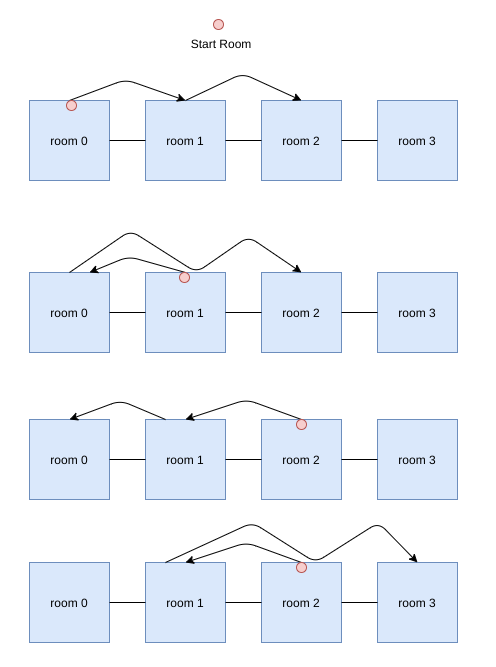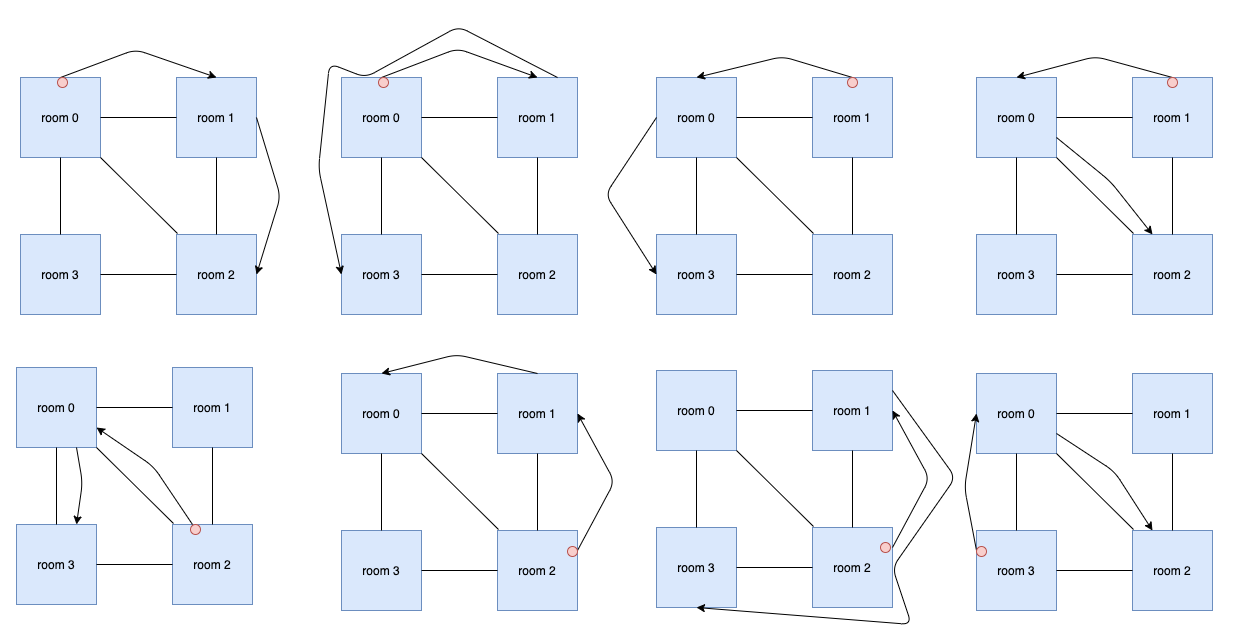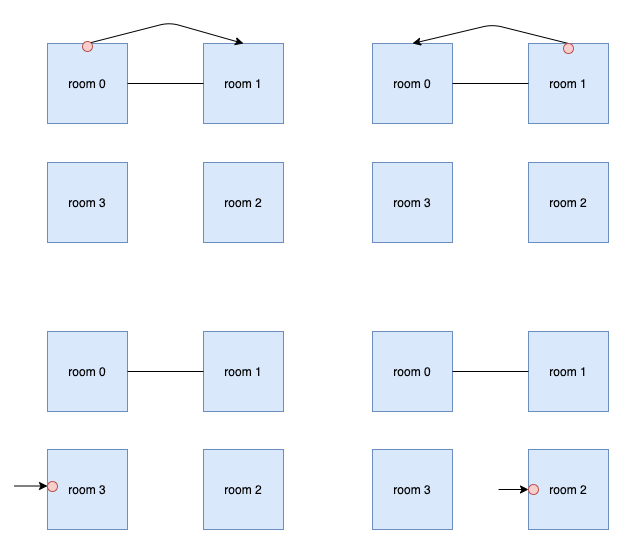시간 제한메모리 제한제출정답맞은 사람정답 비율
서브태스크 참고 (추가 시간 없음) 1024 MB104480.000%

## 문제

Ada lives in a magic country A, and she is studying at Magic University. Today, Ada wants to collect magic points in a special space.

The space has $N$ rooms $(0,1, \cdots ,N-1)$. There are $M$ corridors connecting the rooms. A corridor $j$ connects room $X_j$ and room $Y_j$, meaning you can travel between the two rooms.

The $i$-th room contains $A_i$ magic points and is protected by a magic shield with properties $L_i$ and $R_i$. To enter the $i$-th room, first you need to get to any room adjacent to the $i$-th room (i.e. connected to it by a corridor) through rooms with already broken shields. Then you have to break the shield to this room, but you can break the shield if and only if you have between $L_i$ and $R_i$ magic points, inclusive. After you break the shield, you will enter the room and automatically collect the $A_i$ magic points assigned to this room. The room will not generate new magic points. The room will also not generate a new shield after it is broken, so you can freely go back to every room with already broken shields regardless of the amount of points you have.

Ada starts with $0$ magic points and her goal is to find a way to collect exactly $K$ magic points. She can start in any room, and end in any room. The room she chooses to start in will automatically have its magic shield broken, and she will automatically collect all the magic points from this room.

After inspecting the map of the rooms and corridors, Ada thinks the task is very easy, so she wants to challenge herself with a more difficult task. She wants to know how many unique ways there are to reach the goal. Two ways are different if their unique paths are different. The unique path is the order of rooms in which she broke the shields, e.g.: if you visit the rooms in the order $(1,3,2,1,3,5,3,6)$, the unique path is $(1,3,2,5,6)$.

## 입력

The first line of the input gives the number of test cases, $T$. $T$ test cases follow.

For each test case, the first line contains three integers $N$, $M$, and $K$: the number of rooms, the numbers of corridors, and the numbers of magic points we want to collect, respectively.

The next $N$ lines contain three integers $L_i$, $R_i$, and $A_i$: The magic shield properties $L_i$ and $R_i$ of room $i$, and the number of magic points $A_i$, respectively.

The next $M$ lines contain two integers $X_j$ and $Y_j$: the rooms that are connected by corridor $j$.

## 출력

For each test case, output one line containing Case #x: y, where $x$ is the test case number (starting from 1) and $y$ is the number of ways to collect $K$ magic points.

## 제한

• $1 \le T \le 100$.
• $0 \le M \le \frac{N \times (N-1)}{2}$.
• $0 \le X_j,Y_j \le N-1$.
• $X_j \ne Y_j$.
• Each pair of rooms can be connected by at most one corridor.

## Test Set 1 (15점)

시간 제한: 20 초

• $1 \le N \le 8$.
• $1 \le K \le 100$.
• $0 \le L_i \le R_i \le 50$.
• $1 \le A_i \le 50$.

## Test Set 2 (21점)

시간 제한: 60 초

• $1 \le N\le 15$.
• $1 \le K \le 2 \times 10^9$.
• $0 \le L_i \le R_i \le 10^9$.
• $1 \le A_i \le 10^9$.

## 예제 입력 1

3
4 3 3
1 3 1
1 1 1
2 4 1
2 3 1
0 1
1 2
2 3
4 5 3
1 3 1
1 1 1
2 4 1
2 3 1
0 1
1 2
2 3
3 0
0 2
4 1 2
0 4 1
0 4 1
0 4 2
0 4 2
0 1


## 예제 출력 1

Case #1: 4
Case #2: 8
Case #3: 4


## 힌트

In the first case, there are $4$ different ways. They are:In the second case, there are $8$ different ways. They are:In the third case, there are $4$ different ways. They are:## 채점 및 기타 정보

• 예제는 채점하지 않는다.